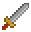# Matrix

The Matrix type encapsulates a 4x4 matrix that can be used in WebGL or for doing affine transformations on Vectors and other Matrices.

The matrix data is stored in column major order, which is the convention for WebGL matrices.

| | | | | | ------- | ------- | -------- | -------- | | data | data | data | data | | data | data | data | data | | data | data | data | data | | data | data | data | data |

## Transforming Vectors

Matrices are an efficient way to transform multiple vectors by the same transformation. Especially if the matrix can be cached.

``````const points = [...];

const mat = Matrix.identity().translate(100, 100).scale(2).rotate(Math.PI / 2);

// transform the points
const transformedPoints = points.map(p => mat.multiply(p));

const inverse = mat.getAffineInverse();

// undo the transform with the inverse
const originalPoints = points.map(p => inverse.multiply(p));
``````

## Multiplying Matrices

Order matters when multiplying matrices `A x B != B x A` . When multiplying matrices the order read in the code

##### Get Involved